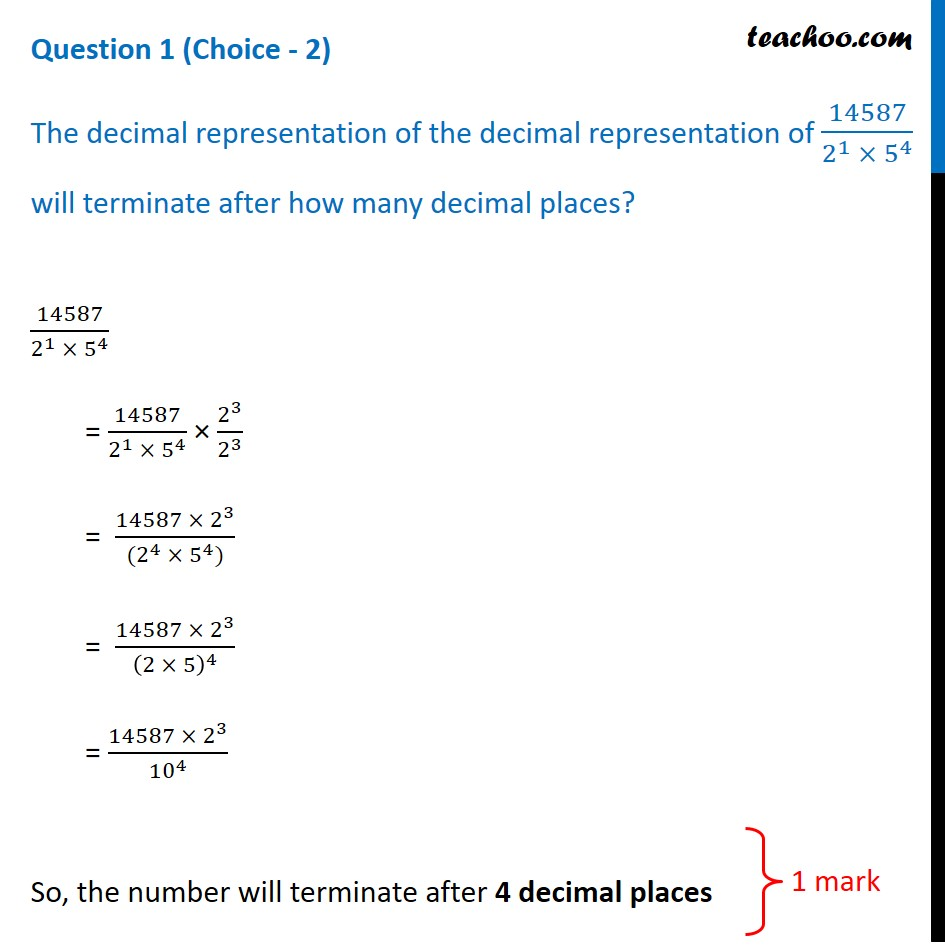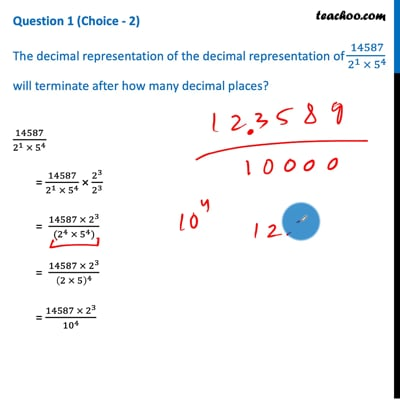CBSE Class 10 Sample Paper for 2021 Boards - Maths Standard

Class 10
Solutions of Sample Papers for Class 10 Boards

## The decimal representation of The decimal representation of 14587/(2 1 × 5 4 ) will terminate after how many decimal places?This video is only available for Teachoo black users

Introducing your new favourite teacher - Teachoo Black, at only ₹83 per month

### Transcript

Question 1 (Choice - 2) The decimal representation of the decimal representation of 14587/(2^1 × 5^4 ) will terminate after how many decimal places?14587/(2^1 × 5^4 ) = 14587/(2^1 × 5^4 ) × 2^3/2^3 = (14587 × 2^3)/((2^4 × 5^4)) = (14587 × 2^3)/(2 × 5)^4 = (14587 × 2^3)/〖10〗^4 So, the number will terminate after 4 decimal places